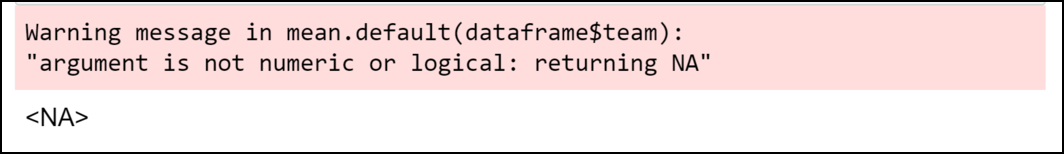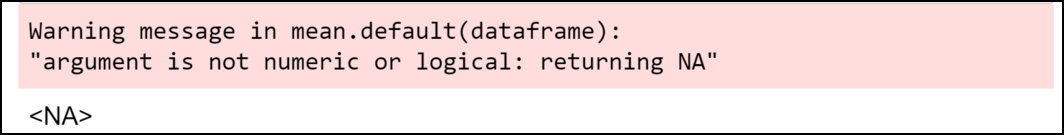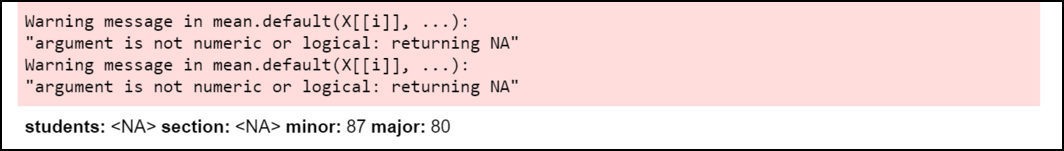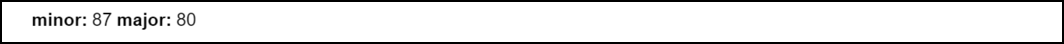GFG App
Open AppBrowser
Continue

# How to Fix in R: Argument is not numeric or logical: returning na

In this article, we are going to see how to fix in R argument is not numeric or logical returning na.

This is the warning message that you may face in R. It takes the following form:

Warning message:

In mean.default(dataframe) : argument is not numeric or logical: returning NA

The R compiler produces such type of error when we try to compute the mean of an object in R but the object contains non-numerical or illogical values. This article focuses on how we can fix this error.

## When this error might occur

Let’s create a data frame first:

## R

 `# Create a data frame ` `dataframe <- ``data.frame``(students=``c``(``'Bhuwanesh'``, ``'Anil'``, ` `                                   ``'Suraj'``, ``'Piyush'``,  ` `                                   ``'Dheeraj'``), ` `                 ``section=``c``(``'A'``, ``'A'``, ``'C'``, ``'C'``, ``'B'``), ` `                 ``minor=``c``(87, 98, 71, 89, 82), ` `                 ``major=``c``(80, 88, 84, 74, 70)) ` ` `  `# Print the dataframe ` `print``(dataframe)`

Output:Output

Now we will try to compute the mean of the values in the section column.

Example:

## R

 `# Create a data frame ` `dataframe <- ``data.frame``(students=``c``(``'Bhuwanesh'``, ` `                                   ``'Anil'``, ``'Suraj'``,  ` `                                   ``'Piyush'``, ``'Dheeraj'``), ` `                 ``section=``c``(``'A'``, ``'A'``, ``'C'``, ``'C'``, ``'B'``), ` `                 ``minor=``c``(87, 98, 71, 89, 82), ` `                 ``major=``c``(80, 88, 84, 74, 70)) ` ` `  `# Try to calculate mean of values in  ` `# section column ` `mean``(dataframe\$team)`

Output:Output

As you can see in the output, the R compiler produces “argument is not numeric or logical: returning NA” as the section has non-numeric values.

Let’s compute the mean of the data frame now:

Example:

## R

 `# Create a data frame ` `dataframe <- ``data.frame``(students=``c``(``'Bhuwanesh'``, ` `                                   ``'Anil'``, ``'Suraj'``, ` `                                   ``'Piyush'``, ``'Dheeraj'``), ` `                 ``section=``c``(``'A'``, ``'A'``, ``'C'``, ``'C'``, ``'B'``), ` `                 ``minor=``c``(87, 98, 71, 89, 82), ` `                 ``major=``c``(80, 88, 84, 74, 70)) ` ` `  `# Try to calculate mean of values  ` `# of the dataframe ` `mean``(dataframe)`

Output:Output

## How to avoid this warning ?

The only way to avoid this warning is to use the mean() function with vectors having numeric values only. For example, In the above example we can calculate the mean of the minor column as it contains numerical values only:

## R

 `# Create a data frame ` `dataframe <- ``data.frame``(students=``c``(``'Bhuwanesh'``, ``'Anil'``,  ` `                                   ``'Suraj'``, ``'Piyush'``,  ` `                                   ``'Dheeraj'``), ` `                 ``section=``c``(``'A'``, ``'A'``, ``'C'``, ``'C'``, ``'B'``), ` `                 ``minor=``c``(87, 98, 71, 89, 82), ` `                 ``major=``c``(80, 88, 84, 74, 70)) ` ` `  `# Try to calculate mean of values stored  ` `# at the column minor in the dataframe ` `mean``(dataframe\$minor)`

Output:Output

## Mean using sapply() function

R provides the sapply() function to compute the mean of values in every column of the data frame.

## R

 `# Create a data frame ` `dataframe <- ``data.frame``(students=``c``(``'Bhuwanesh'``, ``'Anil'``, ` `                                   ``'Suraj'``, ``'Piyush'``,  ` `                                   ``'Dheeraj'``), ` `                 ``section=``c``(``'A'``, ``'A'``, ``'C'``, ``'C'``, ``'B'``), ` `                 ``minor=``c``(87, 98, 71, 89, 82), ` `                 ``major=``c``(80, 88, 84, 74, 70)) ` ` `  `# Try to calculate mean of values stored  ` `# at the column minor in the dataframe ` `sapply``(df, mean, 2)`

Output:Output

As you can see in the output, the R compiler produces the warning message but also calculate the mean values of the columns having numeric values in it. We can avoid the warning completely by explicitly specifying the columns having numeric values.

## R

 `# Create a data frame ` `dataframe <- ``data.frame``(students=``c``(``'Bhuwanesh'``, ``'Anil'``,  ` `                                   ``'Suraj'``, ``'Piyush'``,  ` `                                   ``'Dheeraj'``), ` `                 ``section=``c``(``'A'``, ``'A'``, ``'C'``, ``'C'``, ``'B'``), ` `                 ``minor=``c``(87, 98, 71, 89, 82), ` `                 ``major=``c``(80, 88, 84, 74, 70)) ` ` `  `# Try to calculate mean of values stored  ` `# at the column minor in the dataframe ` `sapply``(dataframe[``c``(``'minor'``, ``'major'``)], mean, 2)`

Output:Output

This time the program compiled successfully without any warning message.

My Personal Notes arrow_drop_up